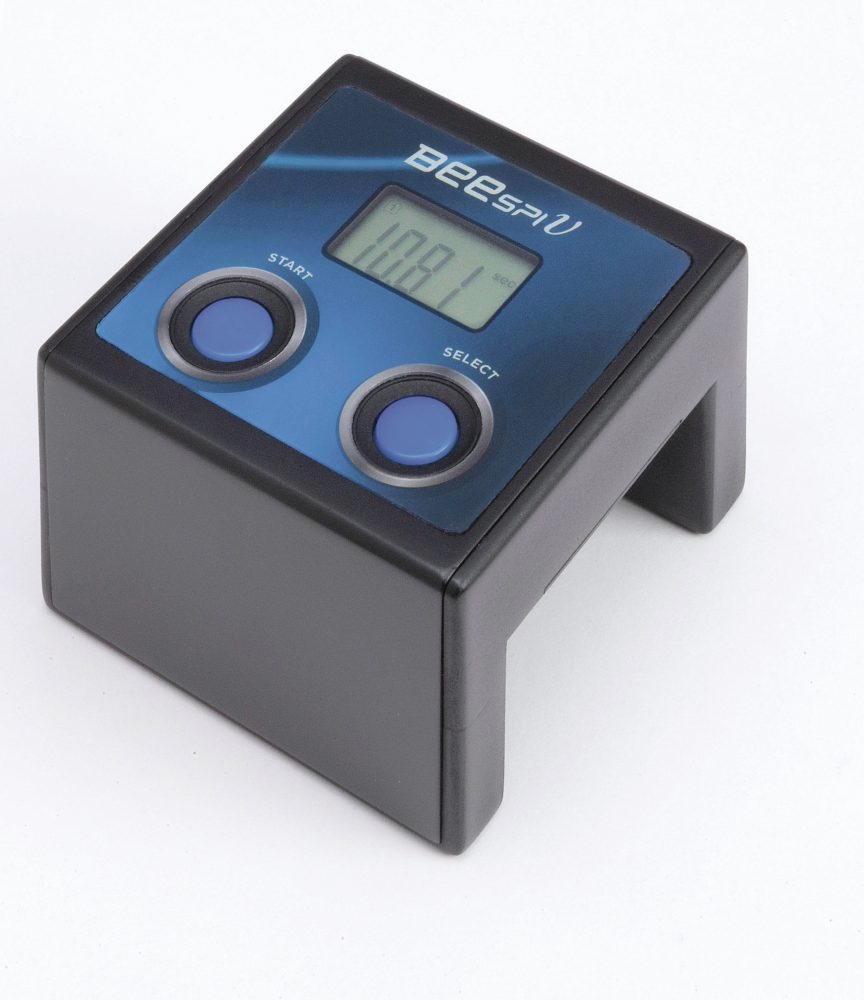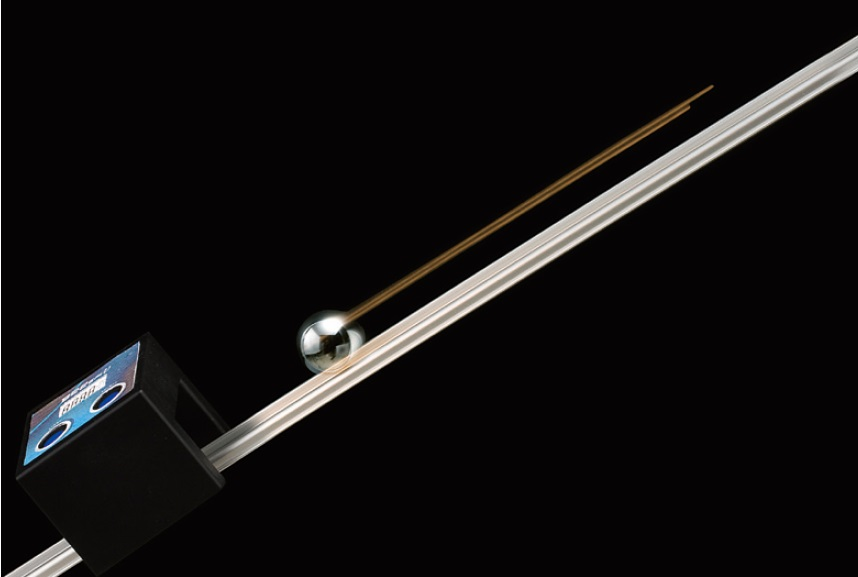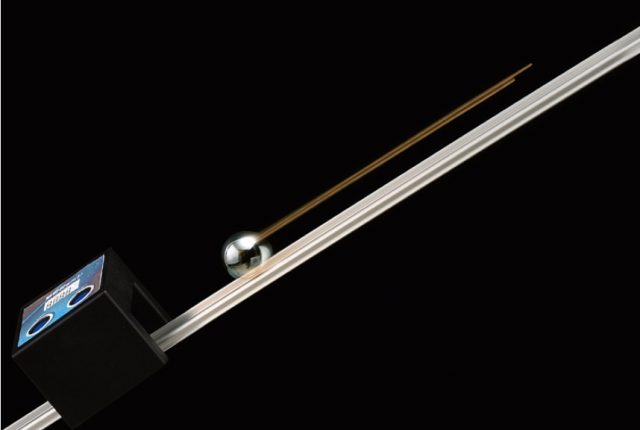# BeeSpi V

S77-1321 S77-1321-10 (Pack of 10pcs)

[Product Description]

Speed Measurement Light Gate

• Compact and easy to use.
• Simple and instant (direct) speed measurement without PC connection.
• Analysis of measured speed data became possible in a STEM way.
• Measured speed can be accurately read from its display which leads to easy calculation of the acceleration (when using x2 BeeSpi V together) or energy (of the measured object).
• Accumulated lap time function.

[Keywords]

• Velocity measurement
• Motion
• Kinetic momentum
• Energy transformation calculation

Click the “Download tab” below for the “Teacher’s Resources” documents such as an instruction manual and teachers’ guide(s) for experiments.Benefit

Specifications

Related Products

MOVIE

[To all users]

• Possible to do experiments at any time and anywhere due to portability of the product.

[To teachers]

• Teachers can spend more time on lecture and demonstration instead of explanation of product usage to students.

[To students]

• Higher efficiency in learning laws of motion through experiments because students no longer need to be familiarized first with use of any software.
• Deeper learning and understanding of relevant topics because students can calculate and make graphs by themselves based on the experiments results in STEM way unlike graph and calculation result automatically generated by data-logging software.
• More time can be spared for analysis of experiments results thanks to almost no time needed for preparation and measurement unlike when using traditional tape-timer or data-logger.
• Students can directly calculate themselves approximate equation close to theoretical equation using measured data from experiments (in line with STEM).
• Law of energy conservation can easily be demonstrated and confirmed because direct measurement of an object’s velocity is possible (the formula of “mgh=1/2 mv2” can be proven when measuring the velocity of a pendulum at the lowest point).
• Speed: 0 to 999.9cm/s, 0 to 99.9m/s, 0 to 99.99km/h
• Lap time: 0 to 99.99sec
• Accumulated Lap Time: 0 to 99.99sec
• Power Source: Two size AAA batteries (sold separately)
• Size: 60 x 60 x 50mm (Inner dimension: 40 x 30mm)
• Weight: 55g (excluding batteries)
• Extra functions: Memory function for saving up to 5 latest measured data

Teacher's Resources

• [EXPERIMENTS] Measurement of pendulum velocity: Portable speed measurement device, BeeSpi v, is recommended product by Narika Corporation for measuring the velocity of weight at the lowest point of the pendulum.

• [EXPERIMENTS] Relationship between velocity of pendulum and the law of conservation of mechanical energy: We will investigate and verify the relationship between motion of pendulum and the law of conservation of mechanical energy by directly measuring the velocity using BeeSpi v.

• [EXPERIMENTS] Relationship between ”Collision Apparatus for Kinetic Energy” and the law of conservation of mechanical energy: Narika’s product, Collision Apparatus for Kinetic Energy (C15-2455-W1), is intended to measure the velocity of a ball launched with certain force and the distance of the objective block moved after collision with the ball.